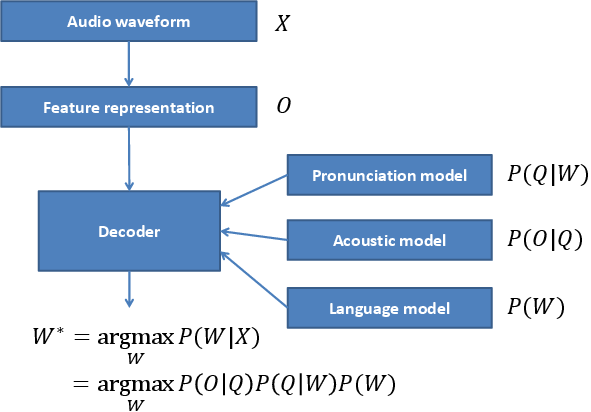# Voice Activity Detection (VAD)¶

## Energy-based¶

A simple energy-based VAD is implemented in bob.kaldi.compute_vad(). The function expects the speech samples as numpy.ndarray and the sampling rate as float, and returns an array of VAD labels numpy.ndarray with the labels of 0 (zero) or 1 (one) per speech frame:

>>> sample = pkg_resources.resource_filename('bob.kaldi', 'test/data/sample16k.wav')
>>> data = bob.io.audio.reader(sample)
>>> print ("Compute VAD"); VAD_labels = bob.kaldi.compute_vad(data.load(), data.rate)
Compute VAD...
>>> print (len(VAD_labels))
317


## DNN-based¶

A Deep Neural Network (DNN), frame-based, VAD is implemented in bob.kaldi.compute_dnn_vad(). Pre-trained DNN on AMI database with headset microphone recordings is used for forward pass of mfcc features. The VAD decision is computed by comparing the silence posterior feature with the silence threshold.

>>> print("Compute DNN VAD"); DNN_VAD_labels = bob.kaldi.compute_dnn_vad(data.load(), data.rate)
Compute DNN VAD...
>>> print (len(DNN_VAD_labels))
317


# Speaker recognition evaluation¶

## MFCC Extraction¶

Two functions are implemented to extract MFCC features bob.kaldi.mfcc() and bob.kaldi.mfcc_from_path(). The former function accepts the speech samples as numpy.ndarray, whereas the latter the filename as str:

1. bob.kaldi.mfcc()

>>> feat = bob.kaldi.mfcc(data.load(), data.rate, normalization=False)
>>> print (feat.shape)
(317, 39)

2. bob.kaldi.mfcc_from_path()

>>> feat = bob.kaldi.mfcc_from_path(sample)
>>> print (feat.shape)
(317, 39)


## UBM training and evaluation¶

Both diagonal and full covariance Universal Background Models (UBMs) are supported, speakers can be enrolled and scored:

>>> # Train small diagonall GMM
>>> diag_gmm_file = tempfile.NamedTemporaryFile()
>>> full_gmm_file = tempfile.NamedTemporaryFile()
>>> print ("ubm train"); dubm = bob.kaldi.ubm_train(feat, diag_gmm_file.name, num_gauss=2, num_gselect=2, num_iters=2)
ubm train...
>>> print ("Train small full GMM"); ubm = bob.kaldi.ubm_full_train(feat, dubm, full_gmm_file.name, num_gselect=2, num_iters=2)
Train...
>>> # Enrollement - MAP adaptation of the UBM-GMM
>>> print ("Enrollement"); spk_model = bob.kaldi.ubm_enroll(feat, dubm)
Enrollement...
>>> print ("GMN scoring"); score = bob.kaldi.gmm_score(feat, spk_model, dubm)
GMN...
>>> print ('%.3f' % score)
0.282


## iVector + PLDA training and evaluation¶

The implementation is based on Kaldi recipe SRE10. It includes ivector extrator training from full-diagonal GMMs, PLDA model training, and PLDA scoring.

>>> plda_file = tempfile.NamedTemporaryFile()
>>> mean_file = tempfile.NamedTemporaryFile()
>>> spk_file = tempfile.NamedTemporaryFile()
>>> test_file = pkg_resources.resource_filename('bob.kaldi', 'test/data/test-mobio.ivector')
>>> features = pkg_resources.resource_filename('bob.kaldi', 'test/data/feats-mobio.npy')
>>> train_feats = numpy.load(features)
>>> test_feats = numpy.loadtxt(test_file)
>>> # Train PLDA model; plda - PLDA model, plda - global mean
>>> print ("Train PLDA"); plda = bob.kaldi.plda_train(train_feats, plda_file.name, mean_file.name)
Train...
>>> # Speaker enrollment (calculate average iVectors for the first speaker)
>>> print ("Speaker enrollment"); enrolled = bob.kaldi.plda_enroll(train_feats, plda)
Speaker...
>>> # Calculate PLDA score
>>> print ("PLDA score"); score = bob.kaldi.plda_score(test_feats, enrolled, plda, plda)
PLDA...
>>> print ('%.4f' % score)
-23.9922


# Deep Neural Networks¶

## Forward pass¶

A forward-pass with pre-trained DNN is implemented in bob.kaldi.nnet_forward(). Output posterior features are returned as numpy.ndarray. First output features of each row (a processed speech frame) contain posteriors of silence, laughter and noise, indexed 0, 1 and 2, respectively. These posteriors are thus used for silence detection in bob.kaldi.compute_dnn_vad(), but might be used also for the laughter and noise detection as well.

>>> nnetfile   = pkg_resources.resource_filename('bob.kaldi', 'test/dnn/ami.nnet.txt')
>>> transfile = pkg_resources.resource_filename('bob.kaldi', 'test/dnn/ami.feature_transform.txt')
>>> feats = bob.kaldi.cepstral(data.load(), 'mfcc', data.rate, normalization=False)
>>> nnetf = open(nnetfile)
>>> trnf = open(transfile)
>>> dnn = nnetf.read()
>>> trn = trnf.read()
>>> nnetf.close()
>>> trnf.close()
>>> print ("NNET forward"); ours = bob.kaldi.nnet_forward(feats, dnn, trn)
NNET...
>>> print (ours.shape)
(317, 43)


# Speech recognition¶

Speech recognition is a processes that generates a text transcript given speech audio. Most of current Automatic Speech Recognition (ASR) systems use the following pipeline:The ASR system has to be first trained. More specifically, its key statistical models:

• Pronunciation model, the lexicon, that associates written and spoken form of words. The lexicon contains words $$W$$ and defines them as sequences of phonemes (the speech sounds) $$Q$$.
• Acoustic model, GMMs or DNNs, that associates the speech features $$O$$ and the spoken words $$Q$$.
• Language model, usually n-gram model, that captures most probably sequences of $$W$$ of a particular language.

The transcript of the input audio waveform $$X$$ is then generated by transformation of $$X$$ to features $$O$$ (for example ceptral features computed by bob.kaldi.cepstral()), and an ASR decoder that outputs the most probable transcript $$W^*$$ using the pre-trained statistical models.

## Acoustic models¶

The basic acoustic model is called monophone model, where $$Q$$ consists just of the phonemes, and consider them contextually independent. The training of such model has following pipeline:

• Model initialization for a given Hidden Markov Model (HMM) structure, usually 3-state left-to-right model.
• Compiling training graphs that compiles Finite State Transducers (FSTs), one for each train utterance. This requires the lexicon, and the word transcription of the training data.
• First alignment and update stage that produces a transition-model and GMM objects for equally spaced alignments.
• Iterative alignment and update stage.
>>> fstfile   = pkg_resources.resource_filename('bob.kaldi', 'test/hmm/L.fst')
>>> topofile = pkg_resources.resource_filename('bob.kaldi', 'test/hmm/topo.txt')
>>> phfile = pkg_resources.resource_filename('bob.kaldi', 'test/hmm/sets.txt')
>>> # word labels
>>> uttid='test'
>>> labels = uttid + ' 27312 27312 27312'
>>> train_set={}
>>> train_set[uttid]=feats
>>> topof = open(topofile)
>>> topo = topof.read()
>>> topof.close()
>>> print ("Train mono"); model = bob.kaldi.train_mono(train_set, labels, fstfile, topo, phfile , numgauss=2, num_iters=2)
Train...
>>> print (model.find('TransitionModel'))
1


## Phone frame decoding¶

Simple frame decoding can by done by finding the indices of the maximum values along the frame axis. The following example performs a forward pass with pre-trained phone DNN, and finds $$argmax()$$ of the output posterior features. Looking at the DNN labels, the phones are decoded per frame.

>>> sample = pkg_resources.resource_filename('bob.kaldi', 'test/data/librivox.wav')
>>> data = bob.io.audio.reader(sample)
>>> print ("Compute dnn phone"); post, labs = bob.kaldi.compute_dnn_phone(data.load(), data.rate)
Compute...
>>> mdecoding = numpy.argmax(post,axis=1) # max decoding
>>> print (labs[mdecoding]) # the last spoken sound of sample is N (of the word DOMAIN)
N